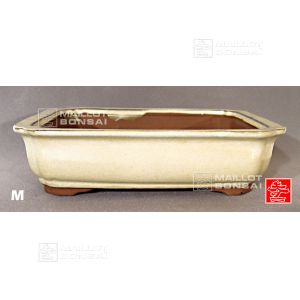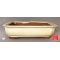##### The Japanese Bonsai specialist
Direct order Contact Help / Services Newsletter# Big rectangular bonsai pot ivory

› Bonsai pots › Rectangular potsref. : 7626

Product unavailable

###### Description

Exterior: 30*25*7.5 cm. Interior: 26*21*5.5 cm. A large ivory glaze bonsai pot in a classic rectangular form.

Buy the matching drip tray Ref 5179

#rectangular 6.1 #bonsai 3.6 #ivory 3.5 #pots 2.4 #exterior 1.8 #interior 1.8 #matching 1.8 #classic 1.7 #tray  1.6 #glaze 1.5

###### Technical description
 shape rectangular color ivory origin China Yixing

Formule
(( ROUND((CHAR_LENGTH(b.article_nom)-CHAR_LENGTH(REPLACE(b.article_nom, 'rectangular', '')))/LENGTH('rectangular')) + ROUND((CHAR_LENGTH(b.article_description)-CHAR_LENGTH(REPLACE(b.article_description, 'rectangular', '')))/LENGTH('rectangular')) ) * 4.1) + (( ROUND((CHAR_LENGTH(b.article_nom)-CHAR_LENGTH(REPLACE(b.article_nom, 'bonsai', '')))/LENGTH('bonsai')) + ROUND((CHAR_LENGTH(b.article_description)-CHAR_LENGTH(REPLACE(b.article_description, 'bonsai', '')))/LENGTH('bonsai')) ) * 3.6) + (( ROUND((CHAR_LENGTH(b.article_nom)-CHAR_LENGTH(REPLACE(b.article_nom, 'ivory', '')))/LENGTH('ivory')) + ROUND((CHAR_LENGTH(b.article_description)-CHAR_LENGTH(REPLACE(b.article_description, 'ivory', '')))/LENGTH('ivory')) ) * 3.5) + (( ROUND((CHAR_LENGTH(b.article_nom)-CHAR_LENGTH(REPLACE(b.article_nom, 'exterior', '')))/LENGTH('exterior')) + ROUND((CHAR_LENGTH(b.article_description)-CHAR_LENGTH(REPLACE(b.article_description, 'exterior', '')))/LENGTH('exterior')) ) * 1.8) + (( ROUND((CHAR_LENGTH(b.article_nom)-CHAR_LENGTH(REPLACE(b.article_nom, 'interior', '')))/LENGTH('interior')) + ROUND((CHAR_LENGTH(b.article_description)-CHAR_LENGTH(REPLACE(b.article_description, 'interior', '')))/LENGTH('interior')) ) * 1.8) + (( ROUND((CHAR_LENGTH(b.article_nom)-CHAR_LENGTH(REPLACE(b.article_nom, 'matching', '')))/LENGTH('matching')) + ROUND((CHAR_LENGTH(b.article_description)-CHAR_LENGTH(REPLACE(b.article_description, 'matching', '')))/LENGTH('matching')) ) * 1.8) + (( ROUND((CHAR_LENGTH(b.article_nom)-CHAR_LENGTH(REPLACE(b.article_nom, 'classic', '')))/LENGTH('classic')) + ROUND((CHAR_LENGTH(b.article_description)-CHAR_LENGTH(REPLACE(b.article_description, 'classic', '')))/LENGTH('classic')) ) * 1.7) + (( ROUND((CHAR_LENGTH(b.article_nom)-CHAR_LENGTH(REPLACE(b.article_nom, 'tray ', '')))/LENGTH('tray ')) + ROUND((CHAR_LENGTH(b.article_description)-CHAR_LENGTH(REPLACE(b.article_description, 'tray ', '')))/LENGTH('tray ')) ) * 1.6) + (( ROUND((CHAR_LENGTH(b.article_nom)-CHAR_LENGTH(REPLACE(b.article_nom, 'glaze', '')))/LENGTH('glaze')) + ROUND((CHAR_LENGTH(b.article_description)-CHAR_LENGTH(REPLACE(b.article_description, 'glaze', '')))/LENGTH('glaze')) ) * 1.5) + (( ROUND((CHAR_LENGTH(b.article_nom)-CHAR_LENGTH(REPLACE(b.article_nom, 'large', '')))/LENGTH('large')) + ROUND((CHAR_LENGTH(b.article_description)-CHAR_LENGTH(REPLACE(b.article_description, 'large', '')))/LENGTH('large')) ) * 1.5)

## Secure payment## Delivery

Our logistic partners :04 74 55 23 48
Pépinière MAILLOT-BONSAÏ
Le Bois Frazy
01990 RELEVANT - FRANCE
on appointment